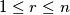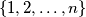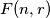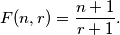### IMO Shortlist 1981 problem 8

Kvaliteta:
Avg: 0,0
Težina:
Avg: 6,0
Take$r$ such that$1\le r\le n$, and consider all subsets of$r$ elements of the set$\{1,2,\ldots,n\}$. Each subset has a smallest element. Let$F(n,r)$ be the arithmetic mean of these smallest elements. Prove that:Izvor: Međunarodna matematička olimpijada, shortlist 1981